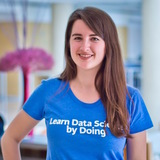Interactive Course

# Time Series Analysis in Python

In this course you'll learn the basics of analyzing time series data.

• 4 hours
• 17 Videos
• 59 Exercises
• 21,417 Participants
• 4,850 XP

### Loved by learners at thousands of top companies:### Course Description

From stock prices to climate data, time series data are found in a wide variety of domains, and being able to effectively work with such data is an increasingly important skill for data scientists. This course will introduce you to time series analysis in Python. After learning about what a time series is, you'll learn about several time series models ranging from autoregressive and moving average models to cointegration models. Along the way, you'll learn how to estimate, forecast, and simulate these models using statistical libraries in Python. You'll see numerous examples of how these models are used, with a particular emphasis on applications in finance.

1. 1

#### Correlation and Autocorrelation

Free

In this chapter you'll be introduced to the ideas of correlation and autocorrelation for time series. Correlation describes the relationship between two time series and autocorrelation describes the relationship of a time series with its past values.

2. #### Autoregressive (AR) Models

In this chapter you'll learn about autoregressive, or AR, models for time series. These models use past values of the series to predict the current value.

3. #### Putting It All Together

This chapter will show you how to model two series jointly using cointegration models. Then you'll wrap up with a case study where you look at a time series of temperature data from New York City.

4. #### Some Simple Time Series

In this chapter you'll learn about some simple time series models. These include white noise and a random walk.

5. #### Moving Average (MA) and ARMA Models

In this chapter you'll learn about another kind of model, the moving average, or MA, model. You will also see how to combine AR and MA models into a powerful ARMA model.

1. 1

#### Correlation and Autocorrelation

Free

In this chapter you'll be introduced to the ideas of correlation and autocorrelation for time series. Correlation describes the relationship between two time series and autocorrelation describes the relationship of a time series with its past values.

2. #### Some Simple Time Series

In this chapter you'll learn about some simple time series models. These include white noise and a random walk.

3. #### Autoregressive (AR) Models

In this chapter you'll learn about autoregressive, or AR, models for time series. These models use past values of the series to predict the current value.

4. #### Moving Average (MA) and ARMA Models

In this chapter you'll learn about another kind of model, the moving average, or MA, model. You will also see how to combine AR and MA models into a powerful ARMA model.

5. #### Putting It All Together

This chapter will show you how to model two series jointly using cointegration models. Then you'll wrap up with a case study where you look at a time series of temperature data from New York City.

### What do other learners have to say?“I've used other sites, but DataCamp's been the one that I've stuck with.”

Devon Edwards Joseph

Lloyd's Banking Group“DataCamp is the top resource I recommend for learning data science.”

Louis Maiden“DataCamp is by far my favorite website to learn from.”

Ronald Bowers

Decision Science Analytics @ USAA##### Rob Reider

Consultant at Quantopian and Adjunct Professor at NYU

Rob is an Adjunct Professor at NYU's Courant Institute where he co-teaches a course on Times Series Analysis and Statistical Arbitrage. He is also currently a Consultant to Quantopian. He has been a Portfolio Manager for over 15 years at Millennium Partners, JPMorgan, and Visium Asset Management. Rob received his Ph.D. in Finance from Wharton.

See More
##### Collaborators
•Lore Dirick

•Nick Solomon